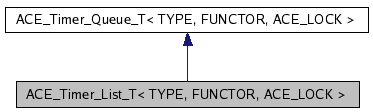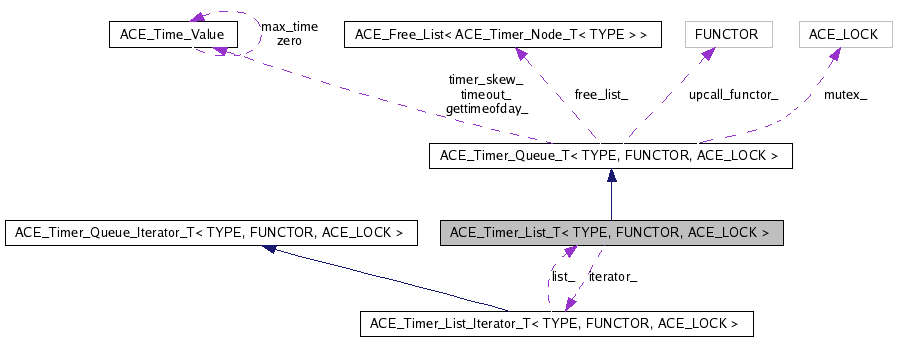# ACE_Timer_List_T< TYPE, FUNCTOR, ACE_LOCK > Class Template Reference

Provides a simple implementation of timers. More...

`#include <Timer_List_T.h>`

Inheritance diagram for ACE_Timer_List_T< TYPE, FUNCTOR, ACE_LOCK >:[legend]
Collaboration diagram for ACE_Timer_List_T< TYPE, FUNCTOR, ACE_LOCK >:[legend]
List of all members.

## Public Types

typedef ACE_Timer_List_Iterator_T<
TYPE, FUNCTOR, ACE_LOCK >
Iterator
Type of iterator.
typedef ACE_Timer_Node_T<
TYPE >
Node
typedef ACE_Timer_Queue_T<
TYPE, FUNCTOR, ACE_LOCK >
Base
Type inherited from.
typedef ACE_Free_List< NodeFreeList

## Public Member Functions

ACE_Timer_List_T (FUNCTOR *upcall_functor=0, FreeList *freelist=0)
virtual ~ACE_Timer_List_T (void)
Destructor.
virtual int is_empty (void) const
True if queue is empty, else false.
virtual const ACE_Time_Valueearliest_time (void) const
virtual int reset_interval (long timer_id, const ACE_Time_Value &interval)
virtual int cancel (const TYPE &type, int dont_call_handle_close=1)
virtual int cancel (long timer_id, const void **act=0, int dont_call_handle_close=1)
virtual ACE_Timer_Queue_Iterator_T<
TYPE, FUNCTOR, ACE_LOCK > &
iter (void)
Returns a pointer to this ACE_Timer_Queue's iterator.
virtual ACE_Timer_Node_T<
TYPE > *
remove_first (void)
Removes the earliest node from the queue and returns it.
virtual void dump (void) const
Dump the state of an object.
virtual void reschedule (ACE_Timer_Node_T< TYPE > *)
virtual ACE_Timer_Node_T<
TYPE > *
get_first (void)
Reads the earliest node from the queue and returns it.

## Private Member Functions

virtual long schedule_i (const TYPE &type, const void *act, const ACE_Time_Value &future_time, const ACE_Time_Value &interval)
void schedule_i (ACE_Timer_Node_T< TYPE > *n, const ACE_Time_Value &exp)
The shared scheduling functionality between schedule() and reschedule().
ACE_Timer_Node_T< TYPE > * find_node (long timer_id) const
void cancel_i (ACE_Timer_Node_T< TYPE > *n)
Shared subset of the two cancel() methods.
void unlink (ACE_Timer_Node_T< TYPE > *n)
ACE_Timer_Node_T< TYPE > * get_first_i (void) const
ACE_Timer_List_T (const ACE_Timer_List_T< TYPE, FUNCTOR, ACE_LOCK > &)
void operator= (const ACE_Timer_List_T< TYPE, FUNCTOR, ACE_LOCK > &)

## Private Attributes

Pointer to linked list of <ACE_Timer_Handles>.
Iteratoriterator_
Iterator used to expire timers.
long id_counter_

## Friends

class ACE_Timer_List_Iterator_T< TYPE, FUNCTOR, ACE_LOCK >
Iterator is a friend.

## Detailed Description

### template<class TYPE, class FUNCTOR, class ACE_LOCK> class ACE_Timer_List_T< TYPE, FUNCTOR, ACE_LOCK >

Provides a simple implementation of timers.

This implementation uses a linked list of absolute times. Therefore, in the average case, scheduling and canceling timers is O(N) (where N is the total number of timers) and expiring timers is O(K) (where K is the total number of timers that are < the current time of day). More clever implementations could use a delta-list, a heap, or timing wheels, etc. For instance, ACE_Timer_Heap is a subclass of ACE_Timer_List that implements a heap-based callout queue. For most applications, the ACE_Timer_Heap will perform substantially faster than the ACE_Timer_List.

## Member Typedef Documentation

template<class TYPE, class FUNCTOR, class ACE_LOCK>
 typedef ACE_Timer_Queue_T ACE_Timer_List_T< TYPE, FUNCTOR, ACE_LOCK >::Base

Type inherited from.

template<class TYPE, class FUNCTOR, class ACE_LOCK>
 typedef ACE_Free_List ACE_Timer_List_T< TYPE, FUNCTOR, ACE_LOCK >::FreeList

template<class TYPE, class FUNCTOR, class ACE_LOCK>
 typedef ACE_Timer_List_Iterator_T ACE_Timer_List_T< TYPE, FUNCTOR, ACE_LOCK >::Iterator

Type of iterator.

template<class TYPE, class FUNCTOR, class ACE_LOCK>
 typedef ACE_Timer_Node_T ACE_Timer_List_T< TYPE, FUNCTOR, ACE_LOCK >::Node

## Constructor & Destructor Documentation

template<class TYPE, class FUNCTOR, class ACE_LOCK>
 ACE_Timer_List_T< TYPE, FUNCTOR, ACE_LOCK >::ACE_Timer_List_T ( FUNCTOR * upcall_functor = `0`, FreeList * freelist = `0` )

Default constructor. upcall_functor is the instance of the FUNCTOR to be used by the list. If upcall_functor is 0, a default FUNCTOR will be created. freelist is the freelist of timer nodes. If 0, then a default freelist will be created.

template<class TYPE, class FUNCTOR, class ACE_LOCK>
 ACE_Timer_List_T< TYPE, FUNCTOR, ACE_LOCK >::~ACE_Timer_List_T ( void ) ` [virtual]`

Destructor.

template<class TYPE, class FUNCTOR, class ACE_LOCK>
 ACE_Timer_List_T< TYPE, FUNCTOR, ACE_LOCK >::ACE_Timer_List_T ( const ACE_Timer_List_T< TYPE, FUNCTOR, ACE_LOCK > & ) ` [private]`

## Member Function Documentation

template<class TYPE, class FUNCTOR, class ACE_LOCK>
 int ACE_Timer_List_T< TYPE, FUNCTOR, ACE_LOCK >::cancel ( long timer_id, const void ** act = `0`, int dont_call_handle_close = `1` ) ` [virtual]`

Cancel the single timer that matches the timer_id value (which was returned from the <schedule> method). If act is non-NULL then it will be set to point to the ``magic cookie'' argument passed in when the timer was registered. This makes it possible to free up the memory and avoid memory leaks. If <dont_call> is 0 then the <functor> will be invoked. Returns 1 if cancellation succeeded and 0 if the <timer_id> wasn't found.

Implements ACE_Timer_Queue_T< TYPE, FUNCTOR, ACE_LOCK >.

template<class TYPE, class FUNCTOR, class ACE_LOCK>
 int ACE_Timer_List_T< TYPE, FUNCTOR, ACE_LOCK >::cancel ( const TYPE & type, int dont_call_handle_close = `1` ) ` [virtual]`

Cancel all timers associated with type. If dont_call_handle_close is 0 then the functor will be invoked. Returns the number of timers cancelled.

Implements ACE_Timer_Queue_T< TYPE, FUNCTOR, ACE_LOCK >.

template<class TYPE, class FUNCTOR, class ACE_LOCK>
 void ACE_Timer_List_T< TYPE, FUNCTOR, ACE_LOCK >::cancel_i ( ACE_Timer_Node_T< TYPE > * n ) ` [private]`

Shared subset of the two cancel() methods.

template<class TYPE, class FUNCTOR, class ACE_LOCK>
 void ACE_Timer_List_T< TYPE, FUNCTOR, ACE_LOCK >::dump ( void ) const` [virtual]`

Dump the state of an object.

Reimplemented from ACE_Timer_Queue_T< TYPE, FUNCTOR, ACE_LOCK >.

template<class TYPE, class FUNCTOR, class ACE_LOCK>
 const ACE_Time_Value & ACE_Timer_List_T< TYPE, FUNCTOR, ACE_LOCK >::earliest_time ( void ) const` [virtual]`

Returns the time of the earlier node in the ACE_Timer_List. Must be called on a non-empty queue.

Implements ACE_Timer_Queue_T< TYPE, FUNCTOR, ACE_LOCK >.

template<class TYPE, class FUNCTOR, class ACE_LOCK>
 ACE_Timer_Node_T< TYPE > * ACE_Timer_List_T< TYPE, FUNCTOR, ACE_LOCK >::find_node ( long timer_id ) const` [private]`

template<class TYPE, class FUNCTOR, class ACE_LOCK>
 ACE_Timer_Node_T< TYPE > * ACE_Timer_List_T< TYPE, FUNCTOR, ACE_LOCK >::get_first ( void ) ` [virtual]`

Reads the earliest node from the queue and returns it.

Implements ACE_Timer_Queue_T< TYPE, FUNCTOR, ACE_LOCK >.

template<class TYPE, class FUNCTOR, class ACE_LOCK>
 ACE_Timer_Node_T< TYPE > * ACE_Timer_List_T< TYPE, FUNCTOR, ACE_LOCK >::get_first_i ( void ) const` [private]`

template<class TYPE, class FUNCTOR, class ACE_LOCK>
 int ACE_Timer_List_T< TYPE, FUNCTOR, ACE_LOCK >::is_empty ( void ) const` [virtual]`

True if queue is empty, else false.

Implements ACE_Timer_Queue_T< TYPE, FUNCTOR, ACE_LOCK >.

template<class TYPE, class FUNCTOR, class ACE_LOCK>
 ACE_Timer_Queue_Iterator_T< TYPE, FUNCTOR, ACE_LOCK > & ACE_Timer_List_T< TYPE, FUNCTOR, ACE_LOCK >::iter ( void ) ` [virtual]`

Returns a pointer to this ACE_Timer_Queue's iterator.

Implements ACE_Timer_Queue_T< TYPE, FUNCTOR, ACE_LOCK >.

template<class TYPE, class FUNCTOR, class ACE_LOCK>
 void ACE_Timer_List_T< TYPE, FUNCTOR, ACE_LOCK >::operator= ( const ACE_Timer_List_T< TYPE, FUNCTOR, ACE_LOCK > & ) ` [private]`

template<class TYPE, class FUNCTOR, class ACE_LOCK>
 ACE_Timer_Node_T< TYPE > * ACE_Timer_List_T< TYPE, FUNCTOR, ACE_LOCK >::remove_first ( void ) ` [virtual]`

Removes the earliest node from the queue and returns it.

Implements ACE_Timer_Queue_T< TYPE, FUNCTOR, ACE_LOCK >.

template<class TYPE, class FUNCTOR, class ACE_LOCK>
 void ACE_Timer_List_T< TYPE, FUNCTOR, ACE_LOCK >::reschedule ( ACE_Timer_Node_T< TYPE > * ) ` [virtual]`

Reschedule an "interval" ACE_Timer_Node_T. This should be private but for now it needs to be public for <ACE_Timer_Hash_T>

Implements ACE_Timer_Queue_T< TYPE, FUNCTOR, ACE_LOCK >.

template<class TYPE, class FUNCTOR, class ACE_LOCK>
 int ACE_Timer_List_T< TYPE, FUNCTOR, ACE_LOCK >::reset_interval ( long timer_id, const ACE_Time_Value & interval ) ` [virtual]`

Resets the interval of the timer represented by timer_id to interval, which is specified in relative time to the current <gettimeofday>. If interval is equal to ACE_Time_Value::zero, the timer will become a non-rescheduling timer. Returns 0 if successful, -1 if not.

Implements ACE_Timer_Queue_T< TYPE, FUNCTOR, ACE_LOCK >.

template<class TYPE, class FUNCTOR, class ACE_LOCK>
 void ACE_Timer_List_T< TYPE, FUNCTOR, ACE_LOCK >::schedule_i ( ACE_Timer_Node_T< TYPE > * n, const ACE_Time_Value & exp ) ` [private]`

The shared scheduling functionality between schedule() and reschedule().

template<class TYPE, class FUNCTOR, class ACE_LOCK>
 long ACE_Timer_List_T< TYPE, FUNCTOR, ACE_LOCK >::schedule_i ( const TYPE & type, const void * act, const ACE_Time_Value & future_time, const ACE_Time_Value & interval ) ` [private, virtual]`

Schedule <type> that will expire at <future_time>, which is specified in absolute time. If it expires then <act> is passed in as the value to the <functor>. If <interval> is != to <ACE_Time_Value::zero> then it is used to reschedule the <type> automatically, using relative time to the current <gettimeofday>. This method returns a <timer_id> that uniquely identifies the the <type> entry in an internal list. This <timer_id> can be used to cancel the timer before it expires. The cancellation ensures that <timer_ids> are unique up to values of greater than 2 billion timers. As long as timers don't stay around longer than this there should be no problems with accidentally deleting the wrong timer. Returns -1 on failure (which is guaranteed never to be a valid <timer_id>).

Implements ACE_Timer_Queue_T< TYPE, FUNCTOR, ACE_LOCK >.

template<class TYPE, class FUNCTOR, class ACE_LOCK>
 void ACE_Timer_List_T< TYPE, FUNCTOR, ACE_LOCK >::unlink ( ACE_Timer_Node_T< TYPE > * n ) ` [private]`

## Friends And Related Function Documentation

template<class TYPE, class FUNCTOR, class ACE_LOCK>
 friend class ACE_Timer_List_Iterator_T< TYPE, FUNCTOR, ACE_LOCK >` [friend]`

Iterator is a friend.

## Member Data Documentation

template<class TYPE, class FUNCTOR, class ACE_LOCK>
 ACE_Timer_Node_T* ACE_Timer_List_T< TYPE, FUNCTOR, ACE_LOCK >::head_` [private]`

Pointer to linked list of <ACE_Timer_Handles>.

template<class TYPE, class FUNCTOR, class ACE_LOCK>
 long ACE_Timer_List_T< TYPE, FUNCTOR, ACE_LOCK >::id_counter_` [private]`

Keeps track of the timer id that uniquely identifies each timer. This id can be used to cancel a timer via the <cancel(long)> method.

template<class TYPE, class FUNCTOR, class ACE_LOCK>
 Iterator* ACE_Timer_List_T< TYPE, FUNCTOR, ACE_LOCK >::iterator_` [private]`

Iterator used to expire timers.

The documentation for this class was generated from the following files:
Generated on Thu Mar 22 07:13:20 2007 for ACE by1.4.7-1